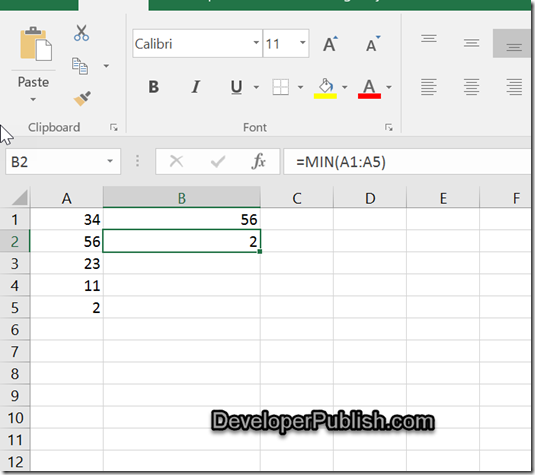How to retreive the highest or lowest value in Microsoft Excel ? - Developer Publish

# How to retreive the highest or lowest value in Microsoft Excel ?

There are times when you might need to select the highest or the lowest value from a range of cells in Microsoft Excel.

# How to get the highest or lowest value in Microsoft Excel ?

There are two formulas in Microsoft Excel that will help you to do it.

• Max – to get the maximum or the largest value from the range of cells.
• Min – to get the minimum or lowest value from the range of cells.

Assume that you have the numbers in the cells A1 to A5 and you want to display the largest value in the cell B1 and the smallest value in the cell B2.

Enter the formula =Max(A1:A5) in the cell B1 and the formula =Min(A1:A5) in the cell B2.